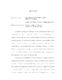## Quantum Detection and Finite Frames##### Files
umi-umd-2245.pdf(479.29 KB)
In quantum mechanics, the definition of a Von Neumann measurement can be generalized using positive-operator-valued measures. This modified definition of a quantum measurement allows one to better distinguish between a set of nonorthogonal quantum states. In this thesis we examine a quantum detection problem, where we have a physical system whose state is limited to be in only one of a finite number of possibilities. These possible states are not necessarily orthogonal. We want to find the best method of measuring the system in order to distinguish which state the system is in. Mathematically, we want to find a positive-operator-valued measure that minimizes the probability of a detection error. It is shown that all tight-frames with frame constant 1 correspond to positive-operator-valued measures. We reformulate the problem in terms of tight-frames that minimize the detection error. In the finite dimensional case, the problem of finding the tight-frame that minimizes the error can be converted into a Hamiltonian system on the group $SO(N)$. The minimum energy solutions of this Hamiltonian system correspond exactly to the tight-frames that minimize the detection error. In this setting, several numerical methods can be applied to give numerical constructions of the desired tight-frames.